Uzuner, S., and Cekmecelioglu, D. (2016). "Comparison of artificial neural networks (ANN) and adaptive neuro-fuzzy inference system (ANFIS) models in simulating polygalacturonase production," BioRes. 11(4), 8676-8685.

#### Abstract

The artificial neural network (ANN) method was used in comparison with the adaptive neuro-fuzzy inference system (ANFIS) to describe polygalacturonase (PG) production by Bacillus subtilis in submerged fermentation. ANN was evaluated with five neurons in the input layer, one hidden layer with 7 neurons, and one neuron in the output layer. Five fermentation variables (pH, temperature, time, yeast extract concentration, and K2HPO4 concentration) served as the input of the ANN and ANFIS models, and the polygalacturonase activity was the output. Coefficient of determination (R2) and root mean square values (RMSE) were calculated as 0.978 and 0.060, respectively for the best ANFIS structure obtained in this study. The R2 and RMSE values were computed as 1.00 and 0.030, respectively for the best ANN model. The results showed that the ANN and ANFIS models performed similarly in terms of prediction accuracy.

#### Full Article

Comparison of Artificial Neural Networks (ANN) and Adaptive Neuro-Fuzzy Inference System (ANFIS) Models in Simulating Polygalacturonase Production

Sibel Uzuner a,* and Deniz Cekmecelioglu b

The artificial neural network (ANN) method was used in comparison with the adaptive neuro-fuzzy inference system (ANFIS) to describe polygalacturonase (PG) production by Bacillus subtilis in submerged fermentation. ANN was evaluated with five neurons in the input layer, one hidden layer with 7 neurons, and one neuron in the output layer. Five fermentation variables (pH, temperature, time, yeast extract concentration, and K2HPO4 concentration) served as the input of the ANN and ANFIS models, and the polygalacturonase activity was the output. Coefficient of determination (R2) and root mean square values (RMSE) were calculated as 0.978 and 0.060, respectively for the best ANFIS structure obtained in this study. The R2 and RMSE values were computed as 1.00 and 0.030, respectively for the best ANN model. The results showed that the ANN and ANFIS models performed similarly in terms of prediction accuracy.

Keywords: Back-propagation network; Artificial intelligence; Polygalacturonase; Adaptive neuro-fuzzy inference system

Contact information: a: Department of Food Engineering, Abant Izzet Baysal University, P. O. Box 14280, Bolu, Turkey; b: Department of Food Engineering, Middle East Technical University, Ankara, Turkey; *Corresponding author: suzuner81@yahoo.com

INTRODUCTION

Enzyme production can be affected by numerous process variables and culture conditions such as pH, temperature, time, minerals, and other fermentation medium components. Thus, fermentation is a complex process due to the possible interaction of these variables. In addition, fermentation medium is crucial for cost-effective enzyme production. To reduce the number of extensive experimentation and learn about complex interactive effects, modeling and optimization is inevitable (Bas and Boyaci 2007; Mingzhi et al. 2009). Predictive models are informative tools for the rapid and cost-effective study of microbial growth, product development, risk assessment, and scientific purposes (Ross 1999). Response surface methodology (RSM) is the most frequently used statistical method in the optimization of fermentation medium parameters. However, RSM does not adequately explain nonlinear models (Ross 1999). To overcome this problem, artificial intelligence-based models such as artificial neural networks (ANN) and adaptive neuro-fuzzy inference system (ANFIS) need to be considered.

ANN is a mathematical algorithm and modeling method that correlates inputs and outputs (Yılmaz 2012). ANFIS is a combination of ANN and fuzzy inference system (FIS). To obtain a better modeling system, ANN can be combined with FIS to improve speed, fault tolerance, and adaptiveness (Jang 1993).

ANN and ANFIS have been successfully applied to model biological systems (Jang and Sun 1995; Bas et al. 2007). ANN is also a popular artificial learning tool in biotechnology and has been used for the optimization of bioprocesses (Monahar and Divakar 2005) and polygalacturonase, protease, laccase, and hydantoinase enzyme production from microorganisms (Nagata and Chu 2003; Dutta et al. 2004; Rekha et al. 2013; Tokatli et al. 2009; Chiranjeevi et al. 2014). Tokatli et al. (2009) compared RSM and ANN models for polygalacturonase activity and biomass production by Aspergillus sojae ATCC 20235, concluding that the ANN model predicted polygalacturonase activity better than the RSM model. In another study, Rekha et al. (2013) successfully used ANN for modeling polygalacturonase production from a newly isolated Thalassopira frigidphilosprofundus. Edupuganti et al. (2014) reported the optimization of alpha-galactosidase production in submerged fermentation by Acinetobacter sp. using feed-forward neural networks. The effect of six different variables including pH, temperature, agitation speed, carbon source (raffinose), nitrogen source (tryptone), and K2HPO4 on enzyme yield was evaluated, and a topology of 6-10-1 for a feed-forward neural network was constructed to determine the interactions between fermentation variables and enzyme yield. Rani et al. (2014) compared RSM and ANN models to enhance the production of phytase by Rhizopus oryzae. The ANN models were also applied to predict and enhance the production of alkaline protease from Bacillus circulans and glutaminase from Bacillus subtilis (Rao et al. 2008; Sathish and Prakasham 2010).

There are a number of studies in which models that are developed based on RSM and ANN use the same experimental design to optimize the fermentation medium. In recent years, a limited number of studies have investigated the possibility of using nonlinear modeling techniques in fermentation processes, including ANN and ANFIS. However, there has not been a comparative study of ANN and ANFIS models for predicting polygalacturonase activity. This is the first report on the prediction of enzyme production using an ANFIS model in comparison with an ANN model. This work focused on predicting the production of polygalacturonase from Bacillus subtilis by ANN and ANFIS models. Several ANN and ANFIS models were developed to predict polygalacturonase activity on the basis of inputs. The accuracy of the estimated data was defined, and the overall prediction ability of these two techniques was compared.

EXPERIMENTAL

Fermentation System

The data used in this study were obtained from submerged fermentation studies with Bacillus subtilis, which was kindly provided by the ARS culture collection, Northern Regional Research Laboratory (NRRL), Peoria, IL, USA. The details concerning the inoculation, fermentation, and measurement of enzyme activity steps are given elsewhere (Uzuner and Cekmecelioglu 2015).

Artificial Neural Network (ANN) Modeling

The input variables used in the ANN models were pH (X1), temperature (X2), time (X3), yeast extract concentration (X4), and K2HPO4 concentration (X5). The ANN model was generated for output variable polygalacturonase (PG) activity (Y1). Several ANNs with different numbers of hidden layer neurons were developed for describing PG production. Input data were randomized into three sets: learning, validation, and testing. Usually, 30% of data are used for testing, and the remaining 70% for training and validation (Mehdizadeh and Movagharnejad 2011). The experimental data included 92 data points. For ANN modeling, the collected experimental data were divided into training (50%), checking (cross-validation) (20%), and testing (validation) (30%) datasets. ANNs were developed using MATLAB R2011a. The first step in neural network modeling is to select the topology of the network. In this network, there are input layer with five inputs, a hidden layer consisting of seven neurons, scalar weights, biases and sigmoid transfer function (logsig, Eq.1) and an output layer including one neuron, scalar weights, a bias, and a linear transfer function (purelin, Eq.2) (Fig 1a).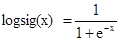(1)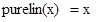(2)

The network training was performed with the function of “trainlm”, which updates weight and bias values according to Levenberg-Marquardt optimization. Trainlm employs the Levenberg-Marquardt backpropagation algorithm that can be expressed as the sum of squares of non-linear real-valued functions. “learngd” was used as the adaption learning function in the learning of the network. learngd is the gradient descent weight and bias learning function. The maximum training epochs were 1000, and the mean square error was 0.0001. The other parameters of the neural network were taken as defaults of the neural network toolbox, MATLAB R2011a.

ANFIS Modeling

Fuzzy systems are classified into three types: Mamdani’s system, Tsukamoto’s system, and Sugeno’s system. Mamdani’s system is the most utilized, whereas Sugeno’s system is more compact and efficient. Moreover, it is the most popular tool for fuzzy modeling (Takagi and Sugeno 1985; Cobaner et al. 2009). Therefore, the Sugeno-type fuzzy system was used in this study. In the ANFIS modeling, the data sets were divided into three groups for training, testing, and validating. For ANFIS modeling, the collected experimental data were divided into training (50%), checking (cross-validation) (20%) and testing (validation) (30%) datasets. The fuzzy logic toolbox of MATLAB R2011a was used for the non-linear modeling of PG activity. The ANFIS model was optimized with a backpropagation algorithm. For selection of the best fit fuzzy model, 3 different ANFIS models that possessed the same number of membership functions (3), different types of membership function for input (Gaussian, triangular shaped, trapezoidal shaped), and similar membership functions for output (linear) were constructed. The maximum learning epochs were 1000, and the mean square error was 0.001. The ANFIS structure of polygalacturonase activity consisted of five layers (Fig. 1).

The first layer of the model consisted of the input variable (pH, temperature, time, yeast extract, and K2HPO4 concentration) membership functions (MFs). The main purpose of this layer was to provide the inputs to the next layer. The second layer was a membership layer and determined the weights for each MF. The fuzzification layer (layer 2) contained 15 nodes and 45 non-linear parameters (Fig. 1). The antecedent rule layer (layer 3) with 251 nodes performed a pre-condition matching process for fuzzy rules. The consequent rule layer (layer 4) with 251 nodes and 1458 linear parameters took the inference of the rules and generated output values. The consequent rule layer (layer 4) was determined using the backpropagation algorithm. The inference rule (output) layer of polygalacturonase activity (layer 5) combined the inputs and transformed the fuzzy classification into a binary result.

a.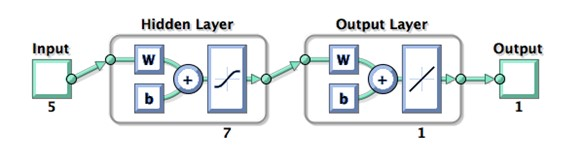b.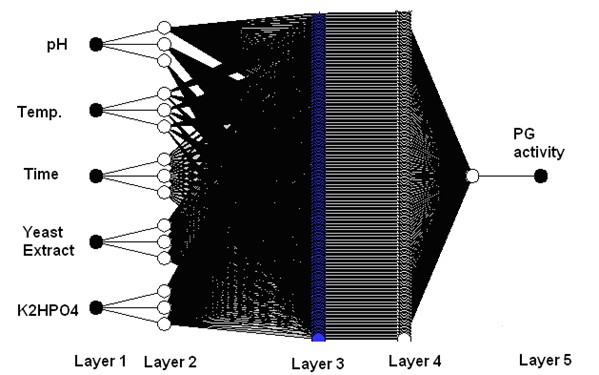Fig. 1. Schematic representation of (a) ANN and (b) ANFIS to simulate polygalacturonase activity

Selection of the Best Model

To compare the constructed models and choose the best one, the performance of the models was measured using common comparison tools in modeling including R2, root mean square error (RMSE, Eq. 3), and mean absolute error (MAE, Eq. 4),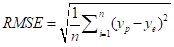(3)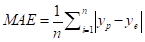(4)

where n was the number of experiments, yp was the predicted value, and ye was the experimental value.

Statistical Analysis

Statistical analyses were carried out to test the significance of different polygalacturonase production. Pairwise comparisons were made by Tukey’s test with a significance level of 0.05.

RESULTS AND DISCUSSION

Predictive Modeling of PG activity using ANN

Effect of architecture and topology on neural network

The selection of network topology in ANN modeling is the key issue. Several parameters such as the number of hidden layers, number of neurons, transfer function, epochs, and learning rate affected the network topology. The number of hidden neurons is one of the most important parameters of ANN modeling. A high number of neurons performs adequately for training data but may fail for testing data (over-fitting), while having too few hidden neurons may result in unsatisfactory convergence (under-fitting) (Tokatli et al. 2009). In this study, the number of neurons in the hidden layer was chosen by trial and error method, by varying the neurons from 3 to 12.

Several ANN models with different network topologies were trained, tested, and evaluated to select the best network topology. The R2, RMSE, and MAE statistics from training and testing data for different ANN topologies is summarized in Table 1.

Table 1. Performance of Different Artificial Neural Network (ANN) Models in Estimation of PG Activity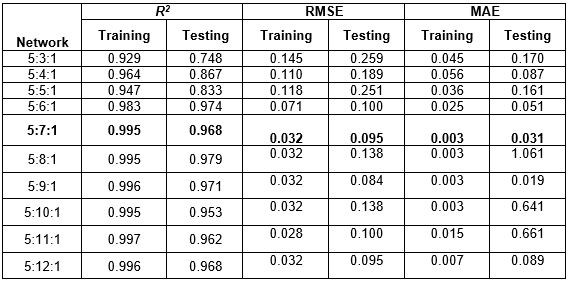Table 1 shows that the 5-7-1 topology was the best, with minimum RMSE and MAE, and maximum R2 values. In the case of training data set, R2 and MAE values were 0.995 and 0.003, respectively, whereas for testing data set, R2 was 0.968 and MAE was 0.031 (Table 1).

The effectiveness of the predictive model results was also compared using RSM for pectinase production from B. subtilis (Uzuner and Cekmecelioglu 2015). The obtained R2 value (0.995) from ANN analysis was higher than the R2 value (0.920) obtained from RSM for the same data. These results indicated that the prediction accuracy of ANN was better than the RSM model for our enzyme production process. Tokatli et al. (2009) performed different ANN topologies to predict polygalacturonase (PG) activity as well with a 5-2-1 network topology (R2=0.84).

Predictive modeling of PG activity using ANFIS

The varying membership function types of input and output were used to evaluate the ANFIS model. The number of membership function (NMF), membership function type of input (MFTI), and membership function type of output (MFTO) for the ANFIS modeling of polygalacturonase activity are reported in Table 2.

Table 2. Performance of Different ANFIS Model in Estimation of PG Activity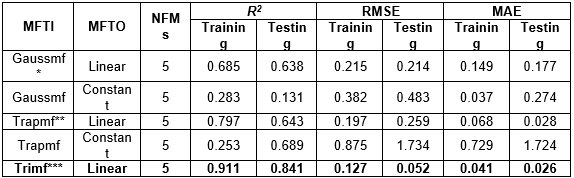*Gaussian membership function,

**Trapezoidal-shaped membership function,

***Triangular-shaped membership function

The R2, RMSE, and MAE values of the constructed models are presented in Table 2. The ANFIS model, including trimf input function type, linear output function type, and 5 membership function number showed the highest accuracy with a high coefficient of determination (R2 = 0.841) and very low RMSE (0.052) and MAE (0.026) values (Table 2). This was higher than other models in Table 2. For the training data set, the coefficient of determination (R2) and RMSE values were 0.911 and 0.127%, respectively. For testing the data set, R2 was 0.841, and RMSE was 0.052% (Table 2).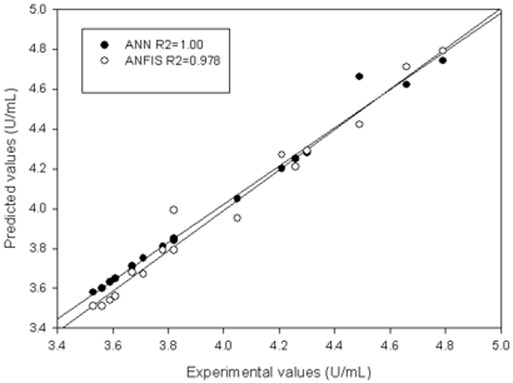Fig. 2. Predicted versus experimental values of PG activity for ANN and ANFIS models

Comparison of ANN and ANFIS models

To evaluate the validity of ANN and ANFIS results, 16 new trials that were not involved in training were used. Figure 2 shows the plot of predicted PG activity by ANN and ANFIS against the experimental values. ANN had a correlation between predicted and actual values for PG production (r = 0.99) in comparison to ANFIS model.

The ANN model exhibited similar predictions with the ANFIS model (Fig. 2). In addition, the best-fit ANN and ANFIS models were derived from the BBD training datasets (Table 1 and 2). After the model was built, the performance of the constructed ANN and ANFIS models was also compared. The comparative statistical values of RMSE and R2 for ANN and ANFIS models are given in Table 3.

Table 3. Summary of the Best Fit ANN and ANFIS Models for PG Activity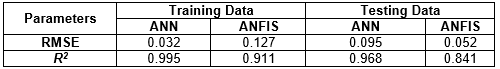Table 4. Validations of Measured Values According to Box-Behnken (BBD) Designs versusPredicted Values by ANN and ANFIS Models for Polygalacturonase Activity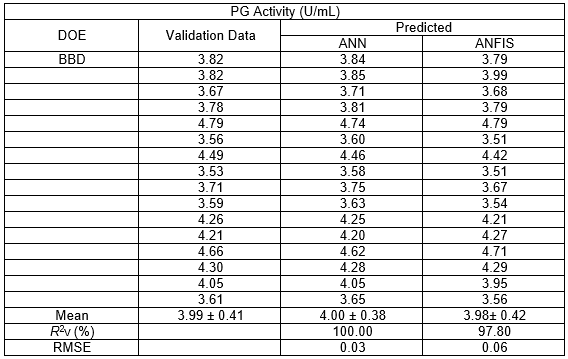Though both models performed well and offered stable responses in prediction, the ANN approach better predicted and fit the testing data. The two best-fit ANN and ANFIS models performed in terms of the independent validation-derived R2 (R2V) and RMSE values for PG production (Table 4). Therefore, the accuracy of the ANN model was higher and had better fitted data than the ANFIS model.

CONCLUSIONS

1. The ANN and ANFIS methods are powerful methods to obtain correlation between input and output in complex nonlinear systems.
2. Trimf and 5-7-1 topologies yielded the best prediction polygalacturonase production using B. subtilis for ANFIS and ANN models, respectively.
3. The best-fit model was identified in the ANN model with the highest R2V (0.10) and lowest RMSE (0.03) values.
4. Prediction of ANN and ANFIS models was similar. Therefore, ANN and ANFIS models can be considered as prediction tools for biological systems such as enzyme activity.

ACKNOWLEDGMENTS

Funding for this study was provided by the METU Research Council (project number BAP-03-14-2011-002) and the Graduate School of Natural and Applied Sciences at the Middle East Technical University, Turkey.

REFERENCES CITED

Bas, D., and Boyaci, I. H. (2007). “Modeling and optimization II: Comparison of estimation capabilities of response surface methodology with artificial neural networks in a biochemical reaction,” J. Food Eng. 78, 846-854. DOI:10.1016/j.jfoodeng.2005.11.025

Bas, D., Dudak, F. C., and Boyaci, I. H. (2007). “Modeling and optimization III: Reaction rate estimation using artificial neural network (ANN) without a kinetic model,” J. Food Eng. 79, 622-628.DOI: 10.1016/j.jfoodeng.2006.02.021.

Chiranjeevi, P. V., Pandian, M. R., and Sathish, T. (2014). “Integration of artificial neural network modeling and genetic algorithm approach for enrichment of laccase production in solid state fermentation by Pleurotus ostreatus,” BioResources 9(2), 2459-2470. DOI: 10.15376/biores.9.2.2459-2470.

Cobaner, M., Unal, B., and Kisi, O. (2009). “Suspended sediment concentration estimation by an adaptive neuro-fuzzy and neural network approaches using hydro-meteorological data,” J. Hydrol. 367, 52-61. DOI:10.1016/j.jhydrol.2008.12.024

Dutta, J. R., Dutta, P. K., and Banerjee, R. (2004). “Optimization of culture parameters for extracellular protease production from a newly isolated Pseudomonas sp. using response surface and artificial neural network models,” Process Biochem. 39, 2193-2198. DOI: 10.1016/j.procbio.2003.11.009

Edupuganti, S., Potumarthi, R., Sathish, T., and Mangamoori, L. N. (2014). “Role of feed forward neural networks coupled with genetic algorithm in capitalizing of intracellular alpha-galactosidase production by Acinetobacter sp.,” BioMed. Res. Int. DOI: 10.1155/2014/361732.

Jang, J. S. R. (1993). “ANFIS: Adaptive network based fuzzy inference system,” IEEE Trans. Syst. Man. Cybern. 23, 665-685. DOI: 10.1109/21.256541

Jang, J. S. R., and Sun, C. T. (1995). “Neuro-fuzzy modeling and control,” Proc. IEEE 83, 378-406.DOI: 10.1109/5.364486

Manohar, B., and Divakar, S. (2005). “An artificial neural network analysis of porcine pancreas lipase catalyzed esterification of anthranilic acid with methanol,” Process Biochem. 40, 3372-3376.DOI: 10.1016/j.procbio.2005.03.045

Mehdizadeh, B., and Movagharnejad, K. (2011). “A comparison between neural network method and semi empirical equations to predict the solubility of different compounds in supercritical carbon dioxide,” Fluid Phase Equilibr. 303, 40-44. DOI:10.1016/j.fluid.2010.12.010.

Mingzhi, H., Ma, Y., Jinquan, W., and Yan, W. (2009). “Simulation of a paper mill wastewater treatment using a fuzzy neural network,” Expert Syst. Appl. 36(3), 5064-5070. DOI: 10.1016/j.eswa.2008.06.006

Nagata, Y., and Chu, K. H. (2003). “Optimization of a fermentation medium using neural networks and genetic algorithms,” Biotechnol. Lett. 25, 1837-1842. DOI: 10.1023/A:1026225526558.

Rani, R., Kumar, S., and Ghosh, S. (2014). “Optimization of aqueous extraction process to enhance the production of phytase by Rhizopus oryzae using response surface methodology coupled with artificial neural network,” Afr. J. Biotechnol. 13(7), 874-883. DOI: 10.5897/AJB2013.11984

Rao, C. S., Sathish, T., Mahalaxmi, M., Laxmi, G. S., Rao, R. S., and Prakasham, R. S. (2008). “Modelling and optimization of fermentation factors for enhancement of alkaline protease production by isolated Bacillus circulans using feed-forward neural network and genetic algorithm,” J. Appl. Microbiol. 104(3), 889-898. DOI: 10.1111/j.1365-2672.2007.03605.x

Rekha, V. P. B., Ghosh, M., Adapa, V., Oh, S., Pulicherla, K. K., and Rao, K. R. S. S. (2013). “Optimization of polygalacturonase production from a newly isolated Thalassopira frigidphilosprofundus to use in pectin hydrolysis: Statistical approach,” BioMed Res. Int. DOI: 10.1155/2013/750187.

Ross, T. (1999). Predictive Food Microbiology Models in the Meat Industry, Meat and Livestock Australia, Sydney, Australia.

Sathish, T., and Prakasham, R. S. (2010). “Enrichment of glutaminase production by Bacillus subtilis RSP-GLU in submerged cultivation based on neural network-Genetic algorithm approach,” J. Chem. Technol. Biotechnol. 85(1), 50-58.DOI: 10.1002/jctb.2267.

Takagi, T., and Sugeno, M. (1985). “Fuzzy identification of system and its applications to modeling and control,” IEEE Trans. Syst. Man. Cybern 15, 116-132.DOI: 10.1109/TSMC.1985.6313399

Tokatli, F., Tari, C., Unluturk, M., and Baysal, N. G. (2009). “Modeling of polygalacturonase enzyme activity and biomass production by Aspergillus sojae ATCC 20235,” J. Ind. Microbiol. Biotechnol. 36, 1139-1148.DOI:10.1007/s10295-009-0595-y.

Uzuner, S., and Cekmecelioglu, D. (2015). “Enhanced pectinase production by optimizing fermentation conditions of Bacillus subtilis growing on hazelnut shell hydrolyzate,” J. Mol. Catal. B: Enzym. 113, 62-67. DOI: 10.1016/j.molcatb.2015.01.003

Yılmaz, M. T. (2012). “Comparison of effectiveness of adaptive neuro-fuzzy inference system and artificial neural networks for estimation of linear creep and recovery properties of model meat emulsions,” J. Texture Stud. 43, 384-399. DOI:10.1111/j.1745-4603.2012.00349.x

Article submitted: May 2, 2016; Peer review completed: June 12, 2016; Revised version received and accepted: August 12, 2016; Published: August 29, 2016.

DOI: 10.15376/biores.11.4.8676-8685